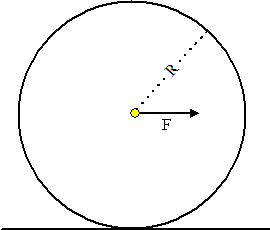Visit the website 123iitjee.manishverma.site for latest posts, courses, admission & more.

###A horizontal force F is applied at the center of mass of a cylindrical object of mass m and radius R, perpendicular to its axis as shown in the figure. The coefficient of friction between the object and the ground is μ. The center of mass of the object has an acceleration a. The acceleration due to gravity is g. Given that the object rolls without slipping, which of the following statement(s) is(are) correct?

(A) For the same F, the value of a does not depend on whether the cylinder is solid or hollow
(B) For a solid cylinder, the maximum possible value of a is 2μg
(C) The magnitude of the frictional force on the object due to the ground is always μmg
(D) For a thin-walled hollow cylinder, $𝑎 = \frac {𝐹}{2𝑚}$

Solution

Torque due to friction $\tau = Rf = I\alpha$

$F-f=ma$

$a=R\alpha$

$\frac {a}{Rf}=\frac {R}{I}$

$\Rightarrow a=\frac {fR^2}{I}$

Now, $f=F-ma=F-f\frac {mR^2}{I}$

$\Rightarrow f=\frac {F}{1+\frac {mR^2}{I}}$

Clearly, frictional force f depends on I, the moment of inertia, option (C) is rejected. Remaining options are about acceleration a.

$a=\frac {fR^2}{I}=\frac {F}{m+\frac {I}{R^2}}$

Since a depends on I, the moment of inertial, option (A) is rejected.

For thin-walled hollow cylinder, $a=\frac {F}{m+\frac {mR^2}{R^2}}=\frac {F}{2m}$ making option (D) correct.

For solid cylinder, $a=\frac {fR^2}{I}=\frac {2fR^2}{mR^2}=\frac {2f}{m}$

Thus, $a_{max}=\frac {2f_{max}}{m}=\frac {2\mu mg}{m}=2\mu g$

So, (B) & (D).

### Sum of the coefficients in the expansion of $(x+y)^n$ ....

If the sum of the coefficients in the expansion of $(x+y)^n$ is 4096, then the greatest coefficient in the expansion is _ _ _ _ . Solution $C_0 + C_1 + C_2 + C_3 + ......................... + C_n =4096$ $\therefore 2^n = 4096 =2^{12}$ $\Rightarrow n = 12$ Greatest coefficient = ${}^{12}{C_6} = 924$